Next: 1.2 Generators, relations and Up: 1 Introduction Previous: 1 Introduction   Contents

## 1.1 Finite type invariants and the fundamental theorem

Summary. A brief introduction to the fundamental theorem and universal finite type invariants. You can find all that (and more) in [BNS].The Fundamental Theorem of Finite Type Invariants1 has a deceptively simple formulation and a surprising number of proofs and partial proofs, each one coming with its own philosophy and employing its own set of tools. The purpose of this article is to further study one family of approaches, the algebraic approaches, not so much as to prove the theorem, for this summit is already multiply climbed, but rather for the mere beauty of these specific paths, for the view from some of the vista points along those, for some new perspectives and insights gained along the way, and, well, o.k., also for an occassional technical advantage over the other approaches. In fact, some of the paths we will take don't even make it all the way to the top, or if they do, they are sometimes obviously non-geodetic, but to find the nearby shorter routes one would have to venture a bit into still unexplored territory. So another reason for the existance of this article is to encourage ourselves, and others, to complete and improve what we already have. Thus quoting from the abstract, this article suggests many live ends'', unfinished problems that don't seem to be dead ends.

In one form, the Fundamental Theorem of Finite Type Invariants, or just the Fundamental Theorem throughout this article, asserts that there exists a universal finite type invariant, an essential invariant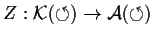. Let us start by defining the terms in this statement, and then, in the further parts of this introduction, we will sketch the algebraic family of approaches (to be followed by full details in Sections 2-4):

•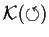is the set of all framed knots. When we draw knots in the plane2 we will always assume the standard blackboard framing, except when using the notationsand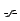, which indicate a right-handed and left-handed framing twists, respectively. Thus for example,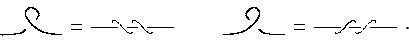•is the usual graded-completed algebra of chord diagrams whose skeleton is a circle, modulo,and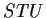relations3: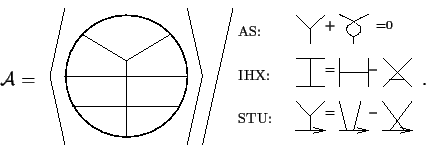• We now come to the only condition thathas to satisfy, that it be essential''. Recall first that any knot invariant with values in an Abelian group can be extended to-singular knots, knots withdouble point, by iterated use of the local formula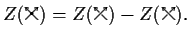Recall also that the symbol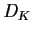of an-singular knotis the degreechord diagram obtained by taking the parameter space of, a circle, and connecting by a chord any pair of points on that circle that are identified in the image: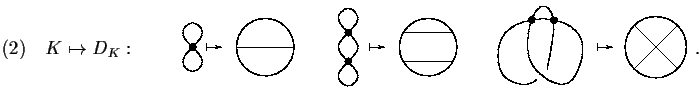The essential'' condition onis that wheneveris an-singular knot,terms of higher degree. (2)

An equivalent formulation of the Fundamental Theorem is that every degreeweight system is theth derivative of some typeinvariant; in particular, it follows that there are lots of finite type invariants, and it reduces the problem of their enumeration to a finite algebro-combinatorial problem at any fixed degree.

#### Footnotes

... Invariants1
Also known as the Fundamental Theorem of Vassiliev Invariants or Kontsevich's Theorem or simply Kontsevich's Integral after the main ingredient of its first proof.
... plane2
As we are often forced to do due to the limitations of our media.
... relations3
If these terms don't seem terribly usual'' to you, you should fix that before proceeding. Being totally unbiased, our favorite reference is [BN1].Next: 1.2 Generators, relations and Up: 1 Introduction Previous: 1 Introduction   Contents
Dror Bar-Natan 2001-07-23